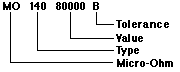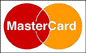How To Order MICRO-OHM Resistors

 Commercial Method: 1. Micro-Ohm Part Number 4. Temperature Coefficient    (if other than standard) 7. Routing 2. Wattage 5. Inductive or    Non-inductive winding 8. Desired Delivery 3. Resistance Value and Tolerance 6. Quantity 9. Certification Requirement Military Method: Example: Type 140 8K ± .1%Resistance The nominal resistance value is a five digit number; the first digits represent the significant figures and the last digit represents the number of zeros to follow (except where preceded by an "R:) When a fractional value of an ohm is required or the value is less than 1000 ohms, the letter "R" is substituted for one of the significant figures to represent the decimal point. The value must be represented by five (5) digits. Examples: Resistance Tolerance: 0.1 ohm = R1000 J = ± 5% 1 ohm = 1R000 F = ± 1% 10 ohms = 10R00 D = ± .5% 100 ohms = 100R0 C = ± .25% 1,000 ohms = 10000 B = ± .1% 10,000 ohms = 10001 A = ± .05% 100,000 ohms = 10002 Q = ± .02% 1,000,000 ohms = 10003 T = ± .01% V = ± .005% Y = ± .001% Federal Stock Code No. 20940

 TO ORDER PRODUCTS E mail: microohm@mail.com Call Toll Free: 1-800-845-5167

 WE ACCEPT